In: Economics

# There are two kinds of factors of production, labor L and capital K, which are only...

There are two kinds of factors of production, labor L and capital K, which are only available in non-negative quantities. There are two ﬁrms that make phones, Apple and Banana. To make qA phones, Apple’s input requirement of (L,K) is given by production function f(L,K) = L0.6K0.2. To make qB phones, Banana’s input requirement of (L,K) is given by production function g(L,K) = L0.75K0.25.
(a) (Time: 3 minutes) How many phones can Apple make with factor bundle (L1,K1) = (1,1)? And how many phones can Banana make with this factor bundle? How many phones can Apple make with factor bundle (L2,K2) = (2,2)? And how many phones can Banana make with this factor bundle?

(b) (Time: 2 minutes) More generally, for any factor bundle (L,K) where L > 1 and L > 1, would you agree that Banana can make more phones than Apple? Explain your answer.

In the case of Apple and Banana, suppose that they both face the same input prices for labor and capital, w and r, respectively, and that the price at which they can sell their output is the same as well, equal to p. Also, assume they are price takers in factor markets as well as the output market. Suppose w = 3 and r = 1.
(c) (Time: 6 minutes) From your answer above, it should be clear that Apple could produce 1 unit of output by using factor bundle (L1,K1). Show why this input bundle is actually cost-minimizing, i.e. the cheapest possible input bundle that ensures an output of 1.

(d) (Time: 2 minutes) Explain why, at output level of 1, Apple’s marginal cost is 5.

Whether or not an output level of 1 maximizes proﬁt will depend upon the price of output. Continue to assume w = 3 and r = 1, and now also suppose p = 5.
(e) (Time: 2 minutes) Explain why output level qA = 1 is proﬁt-maximizing for Apple.

## Solutions

##### Expert Solution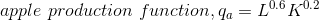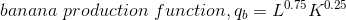Part (a)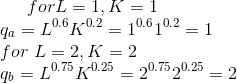Part (b)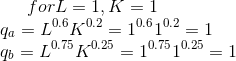if inputs are doubled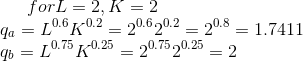Doubling of inputs leads to doubling of output for banana phones. but less than proportionate increase in output is observed for apple phones,

production function for apple phones shows decreasing returns to scale whereas production function for banana phones shows constant returns to scale

Part (c) cost is minimized at point where above condition is fulfilled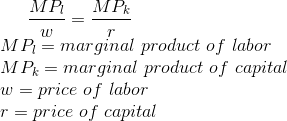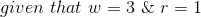we have to find cost minimizing input bundle for an output of 1 unit of apple phones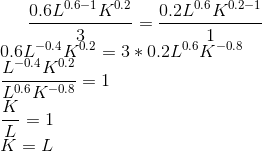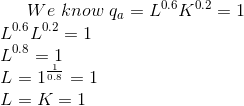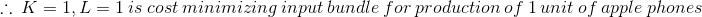Part (d)

we know from the cost minimizing condition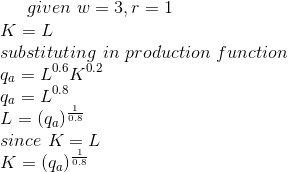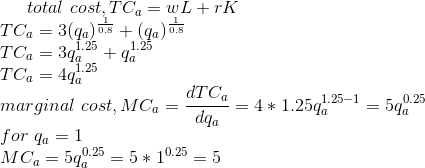Part (e)

given price of an apple phone is 5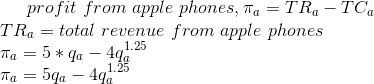maximizing profit by equating first derivative with respect to output to 0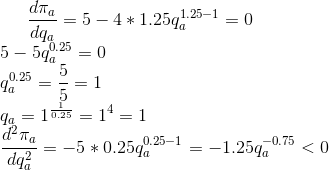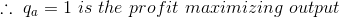## Related Solutions

##### Consider an economy that uses two factors of production, capital (K) and labor (L), to produce...
Consider an economy that uses two factors of production, capital (K) and labor (L), to produce two goods, good X and good Y. In the good X sector, the production function is X = 4KX0.5 + 6LX0.5, so that in this sector the marginal productivity of capital is MPKX = 2KX-0.5 and the marginal productivity of labor is MPLX = 3LX-0.5. In the good Y sector, the production function is Y = 2KY0.5 + 4LY0.5, so that in this sector...
##### The production function has two input, labor (L) and capital (K). The price for L and...
The production function has two input, labor (L) and capital (K). The price for L and K are respectively W and V. q = L + K a linear production function q = min{aK, bL} which is a Leontief production function 1.Calculate the marginal rate of substitution. 2.Calculate the elasticity of the marginal rate of substitution. 3.Drive the long run cost function that is a function of input prices and quantity produced.
##### A firm produces output y using two factors of production (inputs), labour L and capital K....
A firm produces output y using two factors of production (inputs), labour L and capital K. The firm’s production function is ?(?,?)=√?+√?=?12+?12. The wage rate w = 6 and the rental price of capital r = 2 are taken as parameters (fixed) by the firm. a. Show whether this firm’s technology exhibits decreasing, constant, or increasing returns to scale. b. Solve the firm’s long run cost minimization problem (minimize long run costs subject to the output constraint) to derive this...
##### Imagine a firm that only uses capital (K) and labor (L). Use an isocost / isoquant...
Imagine a firm that only uses capital (K) and labor (L). Use an isocost / isoquant diagram to illustrate the firm’s equilibrium input mix for given prices of capital and labor and a given rate of output. Now illustrate what happens if the price of labor falls, and the firm wants to produce the same rate of output. What happens to the cost of production? Compare the relative marginal products of labor and capital (the MRTS) at the two equilibria.
##### Suppose a country’s production function has three factors of production: K—capital, L—the number of workers, and...
Suppose a country’s production function has three factors of production: K—capital, L—the number of workers, and H—human capital. The production function is Y = (K^0.3)(L^0.4)(H^0.3). (a) What is the marginal product of labor? How does human capital affect the MPL? (b) What is the marginal product of human capital? How does human capital affect the MPH? c) What share of total income is paid to labor? What share of total income is paid to human capital? (d) What share of...
##### Capital and labor are the only two inputs for the following production process. Capital is fixed...
Capital and labor are the only two inputs for the following production process. Capital is fixed at 4 units, which costs 50 dollars each unit per day. Workers can be hired for 100 each per day. Complete the following table and plot the marginal cost (MC), average total cost (ATC), average variable cost (AVC), average fixed cost (AFC) on the same graph. The quantity of labor input Total output per day AFC AVC ATC MC    0 0 1 100...
##### 1.  A country’s production function depends on labor (L), physical capital (K), human capital (H), and natural...
1.  A country’s production function depends on labor (L), physical capital (K), human capital (H), and natural resources (N).  When L = 200, K = 10, H = 30, and N = 4, output is 80.  What would output be if L = 700, K = 35, H = 105, and N = 14? A.  30                       B.  60                       C.  240                    D.  280                    E.  320 F.  More than one of A-E is possible                           G.  None of A-G is possible                                                ______ 2.  A nation’s real GDP is 2,000,000 and its GDP deflator is 125.  What is its nominal GDP? A.  1,600,000                                                            B.  1,999,875                        ...
##### Consider a production function of two inputs, labor and capital, given by Q = (√L +...
Consider a production function of two inputs, labor and capital, given by Q = (√L + √K)2. Let w = 2 and r = 1. The marginal products associated with this production function are as follows:MPL=(√L + √K)L-1/2MPK=(√L + √K)K-1/2 a) Suppose the firm is required to produce Q units of output. Show how the cost-minimizing quantity of labor depends on the quantity Q. Show how the cost-minimizing quantity of capital depends on the quantity Q. b) Find the equation...